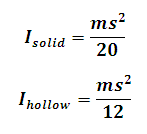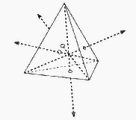# Moment of Inertia of a Tetrahedron Calculator

This Calctown Calculator calculates the Moment of Inertia of a Solid and Hollow Tetrahedron about a Perpendicular axis passing through the centroid.

kg
m

#### Result

kg-m2
kg-m2Click here to view image

where

Ihollow = Moment of Inertia of hollow tetrahedron

Isolid = Moment of Inertia of solid tetrahedron

m = mass of tetrahedron

s = side of tetrahedron